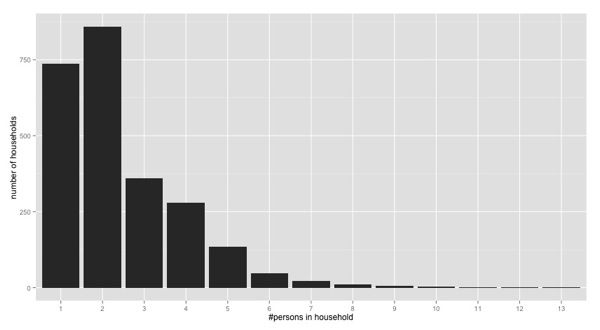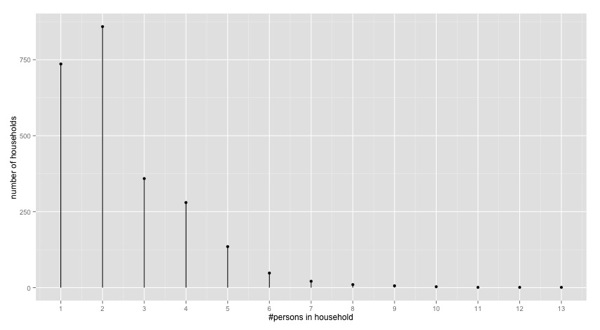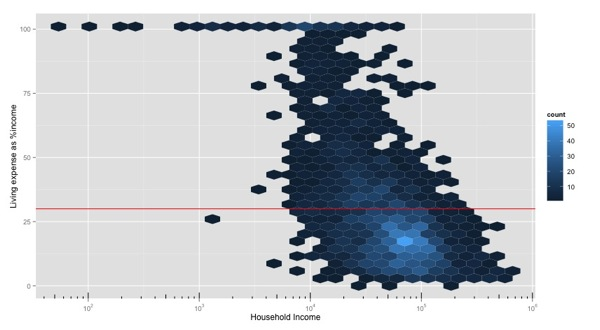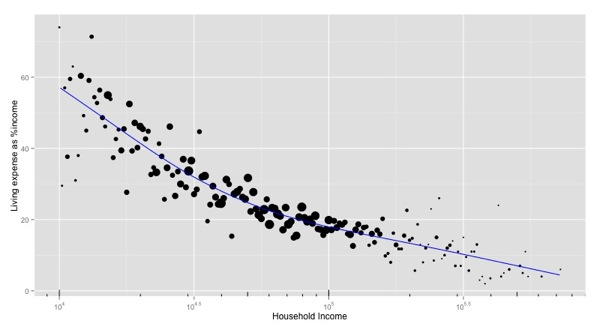# The Extra Step: Graphs for Communication versus Exploration

Visualization is a useful tool for data exploration and statistical analysis, and it’s an important method for communicating your discoveries to others. While those two uses of visualization are related, they aren’t identical.

One of the reasons that I like ggplot so much is that it excels at layering together multiple views and summaries of data in ways that improve both data exploration and communication. Of course, getting at the right graph can be a bit of work, and often I will stop when I get to a visualization that tells me what I need to know — even if no one can read that graph but me. In this post I’ll look at a couple of ggplot graphs that take the extra step: communicating effectively to others.

For my examples I’ll use a pre-treated sample from the 2011 U.S. Census American Community Survey. The dataset is available as an R object in the file `phsample.RData`; the data dictionary and additional information can be found here. Information about getting the original source data from the U.S. Census site is at the bottom of this post.

The file `phsample.RData` contains two data frames: `dhus` (household information), and `dpus` (information about individuals; they are joined to households using the column `SERIALNO`). We will only use the `dhus` data frame.

```library(ggplot2)

# Restrict to non-institutional households
# (No jails, schools, convalescent homes, vacant residences)
hhonly = subset(dhus, (dhus\$TYPE==1) &(dhus\$NP > 0))```

Dotplots for Discrete Distributions

Suppose you want to plot aggregations of the data along discrete numerical values: for example the distribution of household size, or the number of houses bought every year. We’ll plot the distribution of household size in this example. Seems easy, right? A histogram should do it.

```# get the largest household size in the data
maxfam = max(hhonly\$NP, na.rm=T)
breaks=0:maxfam

ggplot(hhonly, aes(x=NP)) + geom_histogram(binwidth=1) +
scale_x_continuous("#persons in household",
breaks=breaks, labels=breaks) +
scale_y_continuous("number of households")```This tells me what I need to know: most households in the U.S. consist of one or two people, and the number falls off from there. For internal data exploration, I would stop here and move on.

But I wouldn’t share this graph with people outside the project. It’s a bit hard to read: the ticks are aligned to the left side of the bars, rather than centered, and because the bars touch, it’s harder to discern the aggregation values that correspond to mid-range household sizes. These problems will be worse when the range of the data is wide, for example if you are aggregating the number of visits to your website at a minute granularity. Reading more than an hour’s worth of that kind of data in this format would be difficult even for internal data exploration, let alone external consumption.

Since the x-values are discrete, you can treat them as factors:

```ggplot(hhonly, aes(x=as.factor(NP))) + geom_bar() +
scale_x_discrete("#persons in household",
breaks=breaks, labels=breaks) +
scale_y_continuous("number of households")```Better. But this will fail if there is a gap in the data, say no households of size 7. In that case, the x-axis would go directly from 6 to 8 — not what you want. For wide data ranges, you will again come up against the problem of the bars touching. Also, you may be of the “bar charts are bad” school of thought. In this case, I don’t think it makes much difference, but there are definitely situations where a dotplot is better.

What works best for external consumption (and internal exploration, too) is something like this:This takes a few extra lines to produce, using the `stat_summary` layer:

```# a dummy function that only returns zero
zero = function(x) {0}

# add a column of all ones to the data frame
# for summation
ggplot(cbind(hhonly, one=1), aes(x=NP, y=one)) +
stat_summary(fun.y="sum", geom="point", size=2) +
stat_summary(fun.ymax="sum", fun.ymin="zero", geom="linerange") +
scale_x_continuous("#persons in household",
breaks=breaks, labels=breaks) +
scale_y_continuous("number of households")```

If you look over Hadley Wickham’s examples of using `stat_summary`, you will see examples of several summary functions that come from the `Hmisc` package. There might be a more succinct way to produce my dotplot using one of these summation functions, but this works well enough for me.

Bubble plots in place of dense scatterplots

I never thought much of bubble plots before, but I saw this variation of them in a blog post from the Computational Story Lab, and I like it a lot. To motivate its use, suppose we want to understand how income affects your ability to find housing within your means. We’ll define “within your means” using the standard financial wisdom that you should spend no more than 30% of your income on housing expenses (rent, or mortgage plus homeowner’s taxes, etc). For income, we’ll use household income. I’ll set up the problem step by step (long-winded, but easier to read).

```# remove households with no (positive) income
filtered = subset(hhonly, hhonly\$HINCP > 0)

expense_frame = with(filtered,
data.frame(np=NP, # household size
hinc = HINCP, # household income
hinc.log10=log10(HINCP),
ocpip=OCPIP, # owner costs as % hinc
grpip=GRPIP)) # gross rent % hinc

# A discretized version of household income, for later
expense_frame\$hinc.bin = 10^(round(expense_frame\$hinc.log10, 2))

# merge the owner and renter expense columns
living_expenses = with(expense_frame,
ifelse(!is.na(ocpip), ocpip,
ifelse(!is.na(grpip), grpip, NA)))

# cost of living (housing) as % household income
expense_frame\$COL_pct = living_expenses

# is the household living beyond its means?
expense_frame\$beyond.means = (living_expenses > 30)

# remove households with no rent or living expenses
expense_frame = subset(expense_frame, !is.na(living_expenses))```

Let’s look at the relative cost of housing as a function of household income. The red line represents the 30% mark; points above it are households living beyond their means.

```library(scales)
ggplot(expense_frame, aes(x=hinc, y=COL_pct)) + geom_point() +
geom_hline(aes(yintercept=30), color="red") +
scale_x_log10("Household Income",
breaks = trans_breaks("log10", function(x) 10^x),
labels = trans_format("log10", math_format(10^.x))) +
annotation_logticks(sides="b") +
scale_y_continuous("Living expense as %income")```Not pretty, but it tells you loud and clear that households making less than \$10,000/year are screwed, and most households making more than about \$125,000/year (that’s 105.1) are doing just fine. Most people live in the range between those two values, and it’s a bit harder to see what’s going on there (and it would be worse with a bigger sample; this sample is only about 2300 households). You can switch `geom_point()` to `geom_hex()` to get a little more insight into the internals of the cloud.A bit more informative, but still not terribly pretty, and hard to explain to people who aren’t used to them; so not the best choice for external consumption. Enter the bubble plot.Each point represents the average value of `y` for a given value of `x` (that’s why we had to discretize household income, earlier). The size of the point represents how many observations went into creating that point: big bubbles mean lots of observations in that income bin, smaller bubbles mean fewer observations. We lose the information about the diffuseness of the data, but we can clearly see the relationship between household income and living expenses, in expectation. This is a plot that is good for public consumption.

Ideally, I’d like to make this plot without creating auxiliary data frames, and perhaps there is a way to do that in the `Hmisc` package, but dealing with extra packages isn’t always worth the effort. So I settled for creating helper frames. First, a function to calculate the average value of `y` over a discrete set of `x` values, along with a weight to indicate how many observations there were for each `x`.

```# This assumes a finite number of x values.
# Return a dataframe
# x: xvalues
# y: mean of y for a given x (like stat_summary(fun.y=mean))
# wt: sqrt of number of datums for that x
#
# use sqrt(count) for the weight, because we perceive area
#
bplot_stats = function(xcol, ycol) {
nrows = length(xcol)
ymeans = aggregate(ycol, by=list(xcol), FUN=mean)
# returns dataframe with colnames (Group.1, x)
xcounts = aggregate(numeric(nrows)+1, by=list(xcol), FUN=sum)
data.frame(x = xcounts\$Group.1, wt = sqrt(xcounts\$x), y=ymeans\$x)
}

# example -- average income per household size (and weight)
bplot_stats(expense_frame\$np, expense_frame\$hinc)

# x        wt         y
# 1   1 26.362853  39645.25
# 2   2 28.965497  69836.16
# 3   3 18.788294  80723.65
# 4   4 16.552945  94756.79
# 5   5 11.489125  88109.98
# 6   6  6.782330  67924.35
# 7   7  4.582576  71825.71
# 8   8  3.162278  66910.00
# 9   9  2.449490  81550.00
# 10 10  1.732051 119666.67
# 11 11  1.000000  96000.00
# 12 12  1.000000  66660.00
# 13 13  1.000000 106700.00```

Notice that the weight is the square root of the number of observations. This is because the weight will dictate the size of the bubble on the plot, and since we perceive area, using the square root of the count will encode relative magnitudes correctly. Now let’s summarize the cost of living data, and plot it.

```# now let's use it on the data
hinc_stats = bplot_stats(expense_frame\$hinc.bin,
expense_frame\$COL_pct)

#
# Remove the legend for wt, because actual magnitude (sqrt of datum count)
# will only confuse the viewer. The qualitative bubble size should be enough
#
ggplot(hinc_stats, aes(x=x,y=y)) + geom_point(aes(size=wt)) +
geom_hline(aes(yintercept=30), color="red") +
scale_x_log10("Household Income",
breaks = trans_breaks("log10", function(x) 10^x),
labels = trans_format("log10", math_format(10^.x))) +
annotation_logticks(sides="b") +
scale_y_continuous("Living expense as %income") +
theme(legend.position="none") # remove the legend for wt```

This produces the bubble plot that we saw above.

[UPDATE Jan 14, 2014: Hadley Wickham has kindly contributed an improved version of this plot. The code is here, and the resulting plot is in the comments, below.]

Bubble Plots and Regression

One of the nice things about this plot is that you see the data the way that a regression algorithm sees the data: as expectations. In particular, even if we restrict ourselves to the income range \$10,000 and above, we can see that the relationship between log income and expected living expense is not linear: the decrease in relative expenses levels off as your income increases. So rather than encoding the relationship with a linear regression, try a GAM (generalized additive model):

```# restrict to households with income > \$10K
gt10K = subset(expense_frame, expense_frame\$hinc.log10 > 4)

# fit a GAM
# we could use library(gam), too, but it has potential
# conflicts with ggplot
library(mgcv)

exp_mod = gam(COL_pct ~ s(hinc.log10), data=gt10K)

summary(exp_mod)  # not shown
# adjusted R-sq of 0.288, and a significant effect from hinc.log10

COL_pct.pred = predict(exp_mod)

# plot it, with the GAM predictions superimposed
mod_stats = bplot_stats(gt10K\$hinc.bin, gt10K\$COL_pct)
tmp = cbind(gt10K, pred=COL_pct.pred)

# the x-axis labels are a little wrong.
# Disregard the labels for 10^4.5 and 10^5.5
ggplot(mod_stats, aes(x=x,y=y)) + geom_point(aes(size=wt)) +
geom_line(data=tmp, aes(x=hinc, y=pred), color="blue") +
scale_x_log10("Household Income",
breaks = trans_breaks("log10", function(x) 10^x),
labels = trans_format("log10", math_format(10^.x))
) +
annotation_logticks(sides="b") +
scale_y_continuous("Living expense as %income") +
theme(legend.position="none")```The fraction of variance explained by the model (28.8%) isn’t great, but it’s not bad, either, when you consider how diffuse the data cloud is.

This bubble plot is a nice way to visualize boolean data (the `beyond.means` column), too. Let’s plot the fraction of the population living beyond their means as a function of household income: summarize using `bplot_stats(gt10K\$hinc.bin, gt10K\$beyond.means)`, and plot as above. The y-axis will represent the fraction of households in each income bin that are spending too much on living expenses.From \$10,000 to \$100,000, the probability of a household living beyonds its means decreases steadily from 100% to near 0% (the decrease looks nearly linear with log income, which implies an exponential falloff with income). The little splattering of households living beyond their means in the \$100,000 – \$150,000 range are probably based in places like San Francisco or New York City, where salaries are high but the cost of housing is even higher.

While the dotplot and the bubble plot are a bit more difficult to create than the more out-of-the-box ggplots, the extra effort is worth it for effective communication of your findings. In fact, if you can encapsulate the plots sufficiently for frequent reuse, they are powerful tools for internal data exploration, as well.

The original census data can be found here, and the corresponding data dictionaries and documentation here. I used a sample of the 2011 data because I happened to have it prepared; the 2012 data is now available on the U.S. Census site as well.

Categories: Coding math programming Statistics Tutorials### Nina Zumel

Data scientist with Win Vector LLC. I also dance, read ghost stories and folklore, and sometimes blog about it all.

### 4 replies ›

1.Brian Slesinsky says:

Hmm, I think I’d actually prefer the scatterplot. You write: “The fraction of variance explained by the model (28.8%) isn’t great, but it’s not bad, either, when you consider how diffuse the data cloud is.”

To me, the scatterplot makes the variation in the data clearer; it shows that, while spending too much on housing is more likely with lower incomes, it’s not uncommon in higher income groups and there’s a lot of variation at each income level.

On the other hand, the bubbleplot looks a bit like a scatterplot but makes the fit look too good due to averaging.

Probably the biggest thing to fix for communication with the public would be to make the scale easier to read; reading incomes using exponential notation on a log scale is not very friendly, and the numbers are printed very small and grey.

1.Nina Zumel says:

Seeing the scatterplot is important when you are working and doing the analysis (that is, internal work), and even in that situation, it can be a problem if the cloud of points is very very dense and the page turns entirely black and hides much of the density structure (I switch to hexbin in that situation). But once the analysis is done, and you are trying to communicate the effect (in expectation), I think the bubble plot is cleaner. In this case, the takeaway message would be something like “the fraction of household income that goes to living expenses drops steadily as income increases, but the decrease begins to level out around \$100,000 [or wherenever the elbow was]”. Mostly I added the gam curve to show that the bubble plot shows expected outcome, just like a regression curve does.

When fitting a model, one should always be clear about how well the model actually fits, of course.

Agreed about the axes. In a real presentation I would have gone ahead and labeled the ticks in non-engineering notation, and made the text bigger/blacker, but setting up the breaks and ticks and adding additional layers to the ggplots added more code that wasn’t central to the code I wanted to show (namely, how to make the dotplot and bubbleplot). The ggplot package is great, but it’s way verbose.

2.Hadley Wickham says:

Great exploration! I’d attack things slightly different in a few places, so I whipped up a quick gist: https://gist.github.com/hadley/8419624

3.Nina Zumel says:

@Hadley Wickham Ah, much better! The labels are much improved this way, as well. Thanks! The resulting plot: